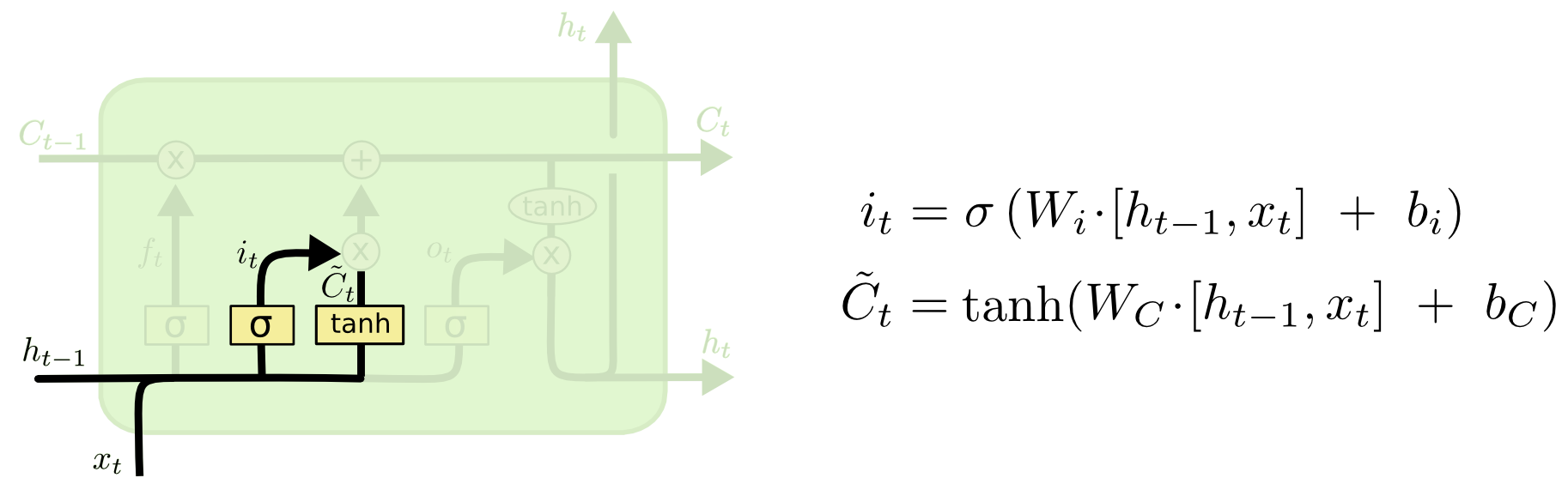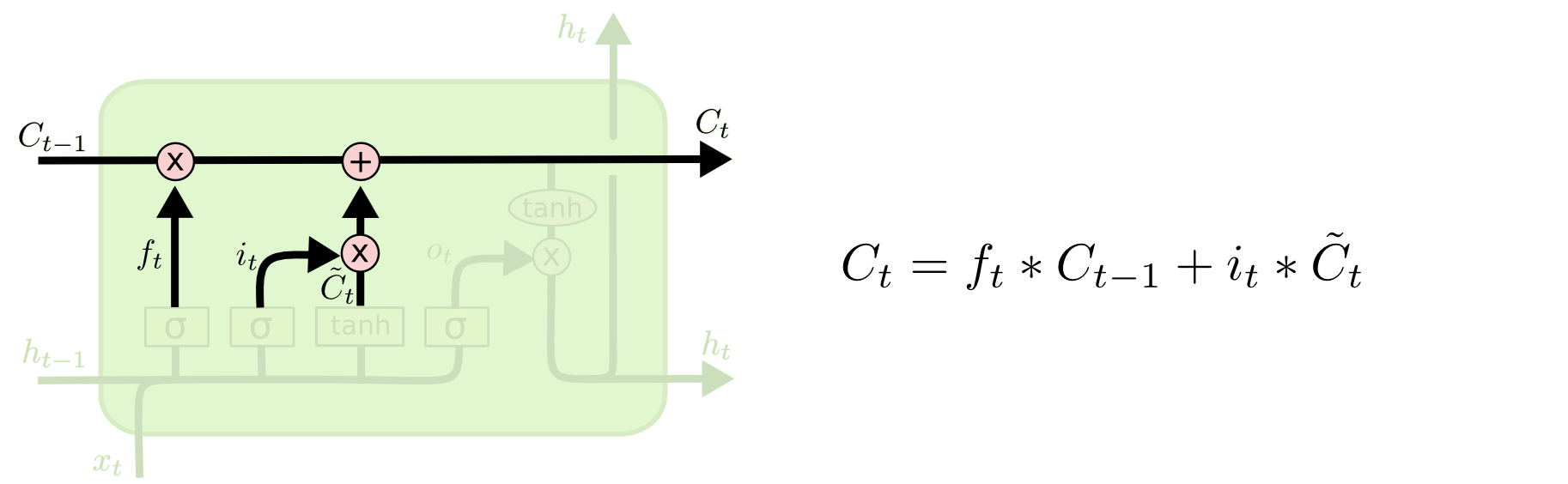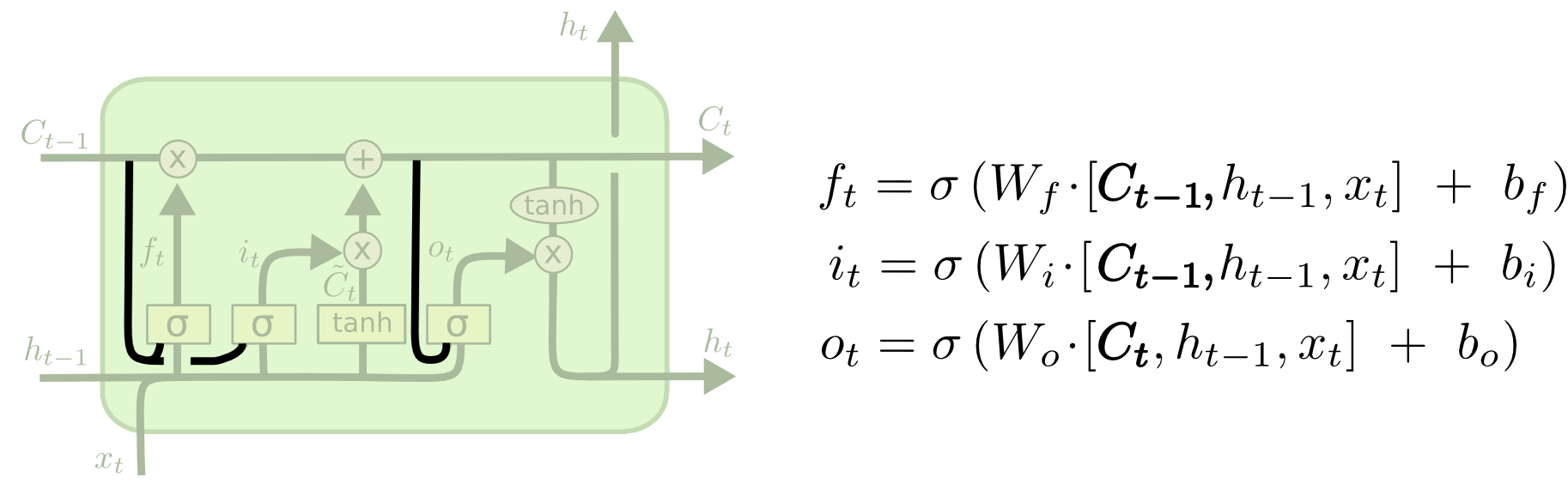# 循环神经网络简介

Posted by lili on

## RNN

1. $x_t$是t时刻的输入。

2. $s_t$是t时刻的隐状态。

它是网络的“记忆”。 $s_t$的计算依赖于前一个时刻的状态和当前时刻的输入： $s_t=f(Ux_t+Ws_{t−1})$。函数f通常是诸如tanh或者ReLU的非线性函数。$s_{−1}$，这是用来计算第一个隐状态，通常我们可以初始化成0。

3. $o_t$是t时刻的输出。

1. 你可以把$s_t$看成是网络的“记忆”。

$s_t$ 捕获了从开始到前一个时刻的所有(感兴趣)的信息，输出 $o_t$ 只基于当前时刻的记忆。不过实际应用中 $s_t$ 很难记住很久以前的信息。

2. 参数共享

传统的神经网络每层使用不同的参数，而RNN的参数(上文的U, V, W)是在所有时刻共享(一样)的。我们每一步都在执行同样的操作，只不过输入不同而已。这种结构极大的减少了我们需要学习的参数。

3. 每一个时刻都有输出

每一个时刻都有输出，但我们不一定都要使用。比如我们预测一个句子的情感倾向是我们只关注最后的输出，而不是每一个词的情感。类似的，我们也不一定每个时刻都有输入。RNN最主要的特点是它有隐状态(记忆)，它能捕获一个序列的信息。

## RNN代码示例

### 数据预处理

data = open('./tiny-shakespeare.txt', 'r').read()  # should be simple plain text file
chars = list(set(data))
data_size, vocab_size = len(data), len(chars)
print('data has %d characters, %d unique.' % (data_size, vocab_size))
char_to_ix = {ch: i for i, ch in enumerate(chars)}
ix_to_char = {i: ch for i, ch in enumerate(chars)}


### 模型超参数和参数定义

# 超参数
hidden_size = 100  # 隐藏层神经元的个数
seq_length = 25  # BPTT时最多的unroll的步数
learning_rate = 1e-1

# 模型参数
Wxh = np.random.randn(hidden_size, vocab_size) * 0.01  # 输入-隐藏层参数
Whh = np.random.randn(hidden_size, hidden_size) * 0.01  # 隐藏层-隐藏层参数
Why = np.random.randn(vocab_size, hidden_size) * 0.01  # 隐藏层-输出层参数
bh = np.zeros((hidden_size, 1))  # 隐藏层bias
by = np.zeros((vocab_size, 1))  # 输出层bias


### 损失函数

def lossFun(inputs, targets, hprev):
"""
inputs,targets都是整数的list
hprev是Hx1的数组，是隐状态的初始值
返回loss，梯度和最后一个时刻的隐状态
"""
xs, hs, ys, ps = {}, {}, {}, {}
hs[-1] = np.copy(hprev)
loss = 0
# forward pass
for t in xrange(len(inputs)):
xs[t] = np.zeros((vocab_size, 1))  # encode in 1-of-k representation
xs[t][inputs[t]] = 1
hs[t] = np.tanh(np.dot(Wxh, xs[t]) + np.dot(Whh, hs[t - 1]) + bh)  # hidden state
ys[t] = np.dot(Why, hs[t]) + by  # unnormalized log probabilities for next chars
ps[t] = np.exp(ys[t]) / np.sum(np.exp(ys[t]))  # probabilities for next chars
loss += -np.log(ps[t][targets[t], 0])  # softmax (cross-entropy loss)
# backward pass: compute gradients going backwards
dWxh, dWhh, dWhy = np.zeros_like(Wxh), np.zeros_like(Whh), np.zeros_like(Why)
dbh, dby = np.zeros_like(bh), np.zeros_like(by)
dhnext = np.zeros_like(hs)
for t in reversed(xrange(len(inputs))):
dy = np.copy(ps[t])
dWhy += np.dot(dy, hs[t].T)
dby += dy
dh = np.dot(Why.T, dy) + dhnext  # backprop into h
dhraw = (1 - hs[t] * hs[t]) * dh  # backprop through tanh nonlinearity
dbh += dhraw
dWxh += np.dot(dhraw, xs[t].T)
dWhh += np.dot(dhraw, hs[t - 1].T)
dhnext = np.dot(Whh.T, dhraw)
for dparam in [dWxh, dWhh, dWhy, dbh, dby]:
np.clip(dparam, -5, 5, out=dparam)  # clip to mitigate exploding gradients
return loss, dWxh, dWhh, dWhy, dbh, dby, hs[len(inputs) - 1]


	# forward pass
for t in xrange(len(inputs)):
xs[t] = np.zeros((vocab_size, 1))  # encode in 1-of-k representation
xs[t][inputs[t]] = 1
hs[t] = np.tanh(np.dot(Wxh, xs[t]) + np.dot(Whh, hs[t - 1]) + bh)  # hidden state
ys[t] = np.dot(Why, hs[t]) + by  # unnormalized log probabilities for next chars
ps[t] = np.exp(ys[t]) / np.sum(np.exp(ys[t]))  # probabilities for next chars
loss += -np.log(ps[t][targets[t], 0])  # softmax (cross-entropy loss)


### sample函数

def sample(h, seed_ix, n):
"""
使用rnn模型生成一个长度为n的字符串
h是初始隐状态，seed_ix是第一个字符
"""
x = np.zeros((vocab_size, 1))
x[seed_ix] = 1
ixes = []
for t in xrange(n):
h = np.tanh(np.dot(Wxh, x) + np.dot(Whh, h) + bh)
y = np.dot(Why, h) + by
p = np.exp(y) / np.sum(np.exp(y))
ix = np.random.choice(range(vocab_size), p=p.ravel())
x = np.zeros((vocab_size, 1))
x[ix] = 1
ixes.append(ix)
return ixes


sample函数会生成长度为n的字符串。一开始x设置为seed_idx：x[seed_idx]=1(这是one-hot表示)，然后和forward类似计算输出下一个字符的概率分布p。然后根据这个分布随机采样一个字符(id) ix，把ix加到结果ixes里，最后用这个ix作为下一个时刻的输入：x[ix]=1。

### 训练

n, p = 0, 0
mWxh, mWhh, mWhy = np.zeros_like(Wxh), np.zeros_like(Whh), np.zeros_like(Why)
smooth_loss = -np.log(1.0 / vocab_size) * seq_length  # loss at iteration 0
while True:
# prepare inputs (we're sweeping from left to right in steps seq_length long)
if p + seq_length + 1 >= len(data) or n == 0:
hprev = np.zeros((hidden_size, 1))  # reset RNN memory
p = 0  # go from start of data
inputs = [char_to_ix[ch] for ch in data[p:p + seq_length]]
targets = [char_to_ix[ch] for ch in data[p + 1:p + seq_length + 1]]

# sample from the model now and then
if n % 1000 == 0:
sample_ix = sample(hprev, inputs, 200)
txt = ''.join(ix_to_char[ix] for ix in sample_ix)
print('----\n %s \n----' % (txt,))

# forward seq_length characters through the net and fetch gradient
loss, dWxh, dWhh, dWhy, dbh, dby, hprev = lossFun(inputs, targets, hprev)
smooth_loss = smooth_loss * 0.999 + loss * 0.001
if n % 1000 == 0:
print('iter %d, loss: %f' % (n, smooth_loss))  # print progress

for param, dparam, mem in zip([Wxh, Whh, Why, bh, by],
[dWxh, dWhh, dWhy, dbh, dby],
[mWxh, mWhh, mWhy, mbh, mby]):
mem += dparam * dparam
param += -learning_rate * dparam / np.sqrt(mem + 1e-8)  # adagrad update

p += seq_length  # move data pointer
n += 1  # iteration counter


## LSTM/GRU

### 长距离依赖(Long Term Dependency)问题

RNN最有用的地方在于它(可能)把之前的信息传递到当前时刻，比如在理解一个视频的当前帧时利用之前的帧是非常有用的。如果RNN可以做到这一点，那么它会非常有用。但是它能够实现这个目标吗？

### Long Short Term Memory(LSTM) 基本概念

LSTM是一种特殊的RNN网络，它使用门(Gate)的机制来解决长距离依赖的问题。回顾一下，所有的RNN都是如下图所示的结构，把RNN看成一个黑盒子的话，它会有一个“隐状态”来“记忆”一些重要的信息。当前时刻的输出除了受当前输入影响之外，也受这个“隐状态”影响。并且在这个时刻结束时，除了输出之外，这个“隐状态”的内容也会发生变化——它“记忆”了新的信息同时有“遗忘”了一些旧的信息。

LSTM也是这样的结构，但相比于原始的RNN，它的内部结构更加复杂。普通的RNN就是一个全连接的层，而LSTM有四个用于控制”记忆”和运算的门，如下图所示。

### LSTM核心思想

LSTM除了有普通RNN的隐状态$h_t$之外还有一个Cell状态$C_t$，它可以从上一个时刻直接通到下一个时刻的（后面会介绍修改它的操作），所以以前的重要记忆可以很容易保存下来，如下图所示，图上从$C_{t-1}$到$C_t$存在直接的通道。图：LSTM Cell State的通道

### LSTM门的细节图：LSTM的输入门 　 接着把上一个时刻未遗忘的信息$C_{t-1} \times f_t$和当前时刻候选$\tilde{C}_t$累加得到新的$C_t$，如下图：$C_t=C_{t-1} \times f_t +\tilde{C}_t$。图：LSTM计算t时刻的$C_t$

$\begin{split} & o_t=\sigma(W_o · [h_{t-1}, x_t]+b_o \\ & h_t=o_t * tanh(C_t) \end{split}$

### LSTM的变种图：有peephole连接的LSTM

$\begin{split} & z_t=\sigma(W_z \times [h_{t-1}, x_t]) \\ & r_t=\sigma(W_r \times [h_{t-1}, x_t]) \\ & \tilde{h}_t=tanh(W \times [r_t * h_{t-1}, x_t]) \\ & h_t=(1-z_t)*h_{t-1}+z_t * \tilde{h}_t \end{split}$